+
Substitution in multi-step linear equations
Solving Linear Equations and Inequalities
0
of 0 possible points

# Substitution in multi-step linear equations

Author: Sophia Tutorial
##### Description:

Use the Substitution Property of Equality to rewrite an expression for another variable.

(more)

Sophia’s self-paced online courses are a great way to save time and money as you earn credits eligible for transfer to many different colleges and universities.*

No credit card required

37 Sophia partners guarantee credit transfer.

299 Institutions have accepted or given pre-approval for credit transfer.

* The American Council on Education's College Credit Recommendation Service (ACE Credit®) has evaluated and recommended college credit for 33 of Sophia’s online courses. Many different colleges and universities consider ACE CREDIT recommendations in determining the applicability to their course and degree programs.

Tutorial
what's covered
1. Substitution Property of Equality
2. Substituting Expressions into Equations
3. Substituting to Solve an Equation

# 1. Substitution Property of Equality

The Substitution Property of Equality allows us to swap or substitute equivalent quantities in expressions and equations. Let's take a look at a basic example of substitution.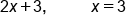Substitute 3 in for x, because they are equal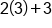Evaluate 2(3)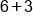Add 6 and 3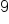Our Solution

# 2. Substituting Expressions in Equations

Sometimes, we are given an expression for the variable, rather than a single value. We can still use the Substitution Property of Equality to simplify expressions and solve equations. Most often, this requires distribution after substituting, in order to simplify the equation or expression. This is illustrated in the example below: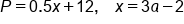Substitute 3a-2 in for x, because they are equal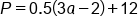Distribute (.05) into (3a-2)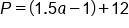Combine like terms -1 and 12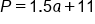Our Solution

hint
If there is a coefficient in front of the variable that is substituted with an expression, it will require that we distribute it into the newly substituted expression in order to simplify.

# 3. Substituting to Solve an Equation

Let's apply the concept of substitution to solve an equation. Suppose you sell gift bags from a kiosk at a local strip mall. Each gift bag costs \$7, and you received \$15 in tips for the day. We can represent your profit with the equation: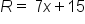, where R is revenue, and x is the number of gift bags sold. (7 is multiplied by x to represent revenue from sales, and 15 is added to account for the tips.)

You figure that you averaged 8 sales per hour, and at the end of the day, a customer bought 10 of them for a party she is attending. We can represent the number of gift bags sold by the equation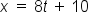, where x is the number of gift bags sold, and t is time in hours. (8 is multiplied by t to represent 8 bags sold each hour, and we add 10 to account for the customer who bought 10 for her party.)

Let's take a look at our equations: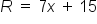How long did it take to generate \$253 in revenue? Notice that we can substitute \$253 in for R, but we want to solve for t, time. One method would be to solve for x, and then substitute that value in order to solve for t. Another method involves making all necessary algebraic substitutions first, and then solving a simplified equation.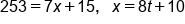Substitute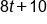in for x, because they are equal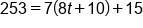Distribute 7 into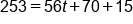Combine like terms 70 and 15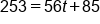Subtract 85 from both sides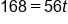Divide by 56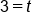Our Solution

This means that \$253 was generated after 3 hours of selling gift bags. Not bad!

summary
The substitution property of equality states that we can substitute an expression that is equal to some variable into another equation or expression containing that same variable. After you substitute, you can simplify and/or solve the equation.

Rating